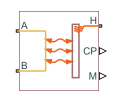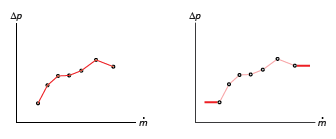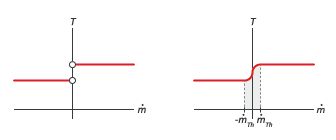# Simple Heat Exchanger Interface (TL)

Thermal interface between a thermal liquid and its surroundings

•Libraries:
Simscape / Fluids / Fluid Network Interfaces / Heat Exchangers / Fundamental Components

## Description

The Simple Heat Exchanger Interface (TL) block models the pressure drop and temperature change in a thermal liquid as it traverses the length of a thermal interface such as that provided by a heat exchanger. Heat transfer across the thermal interface is ignored. See the composite block diagram of the Heat Exchanger (TL-TL) block for an example showing how to combine the two blocks.

The pressure drop is calculated as a function of mass flow rate from tabulated data specified at some reference pressure and temperature. The calculation is based on linear interpolation if the mass flow rate is within the bounds of the tabulated data and on nearest-neighbor extrapolation otherwise. In other words, neighboring data points connect through straight-line segments, with those at the mass flow rate bounds extending horizontally outward.

Linear interpolation (left) and nearest-neighbor extrapolation (right)The block calculations rely on the states and properties of the fluid—temperature, density, and specific internal energy—at the entrance to the thermal interface. The entrance changes abruptly from one port to the other during flow reversal, introducing discontinuities in the values of these variables. To eliminate these discontinuities, the block smooths the affected variables at mass flow rates below a specified threshold value.

Smoothing of entrance temperature below mass flow rate threshold### Mass Balance

Mass can enter and exit the thermal interface through ports A and B. The volume of the interface is fixed but the compressibility of the fluid means that the mass inside the interface can change with pressure and temperature. Whether compressibility is factored into the block calculations depends on the setting of the Thermal Liquid dynamic compressibility parameter in the Effects and Initial Conditions tab:

where:

• $\stackrel{˙}{m}$* are the mass flow rates in through the thermal liquid ports.

• p is the internal fluid pressure.

• T is the internal fluid temperature.

• ɑ is the isobaric thermal expansion coefficient.

• β is the isothermal bulk modulus.

• ρ is the internal fluid density.

• V is the internal fluid volume.

If the Thermal Liquid dynamic compressibility parameter is set to `Off`, the fluid is treated as incompressible and the mass flow rate in through one thermal liquid port must exactly equal that out through the other thermal liquid port. The rate of mass accumulation is, in this case, zero.

### Energy Balance

Energy can enter and exit the thermal interface in two ways: with fluid flow through ports A and B and with heat flow through port H. No work is done on or by the fluid inside the interface. The rate of energy accumulation in the internal fluid volume of the interface must therefore equal the sum of the energy flow rates through all three ports:

`$\frac{\partial E}{\partial p}\frac{dp}{dt}+\frac{\partial E}{\partial T}\frac{dT}{dt}={\varphi }_{\text{A}}+{\varphi }_{\text{B}}+{Q}_{\text{H}},$`

where:

• E is the total energy in the internal fluid volume of the thermal interface.

• ϕ* are the energy flow rates in through the thermal liquid ports.

• Q is the heat flow rate in through the thermal port.

### Momentum Balance

The pressure drop calculation is based entirely on tabulated data that you specify. The causes of the pressure drop are ignored, except in the effects that they might have on the specified data. The overall pressure drop from one thermal liquid port to the other is calculated from the individual pressure drops from each thermal liquid port to the internal fluid volume:

`${p}_{\text{A}}-{p}_{\text{B}}=\Delta {p}_{\text{A}}-\Delta {p}_{\text{B}},$`

where:

• p* are the fluid pressures at the thermal liquid ports.

• Δp* are the pressure drops from the thermal liquid ports to the internal fluid volume:

`$\Delta {p}_{*}={p}_{*}-p,$`

with p as the pressure in the internal fluid volume.

The tabulated data is specified at a reference pressure and temperature from which a third reference parameter, the reference density, is calculated. The ratio of the reference density to the actual port density serves as a correction factor in the individual pressure drop equations, each defined as:

`$\Delta {p}_{*}=\Delta p\left({\stackrel{˙}{m}}_{*}\right)\frac{{\rho }_{\text{R}}}{{\rho }_{*}},$`

where:

• Δp($\stackrel{˙}{m}$) is the tabulated pressure drop function.

• ρ* are the fluid densities at the thermal liquid ports.

The asterisk denotes the thermal liquid port (A or B) at which a parameter or variable is defined. Subscript R denotes a reference value. The density at the interface entrance is smoothed below the mass flow rate threshold by introducing a hyperbolic term ɑ:

`${\rho }_{*,\text{smooth}}={\rho }_{*}\left(\frac{1+\alpha }{2}\right)+\rho \left(\frac{1-\alpha }{2}\right),$`

where ρsmooth is the smoothed density at the entrance port, ρ* is the unsmoothed density at the same port, and ρ is the density in the internal fluid volume. The hyperbolic smoothing term is defined as:

`$\alpha =\text{tanh}\left(4\frac{{\stackrel{˙}{m}}_{\text{avg}}}{{\stackrel{˙}{m}}_{\text{th}}}\right),$`

where $\stackrel{˙}{m}$avg is the average of the mass flow rates through the thermal liquid ports and $\stackrel{˙}{m}$th is the mass flow rate threshold specified in the block dialog box. This threshold determines the width of the mass flow rate region over which to smooth the fluid density. The average mass flow rate is defined as:

`${\stackrel{˙}{m}}_{\text{avg}}=\frac{{\stackrel{˙}{m}}_{\text{A}}+{\stackrel{˙}{m}}_{\text{B}}}{2}$`

## Ports

### Output

expand all

Isobaric specific heat of the thermal liquid in the internal fluid volume of the thermal interface.

Mass flow rate of the thermal liquid in the interior of the thermal interface. The output signal is positive when the flow rate is directed from port A to port B and negative otherwise.

### Conserving

expand all

Opening through which thermal liquid can enter and exit the thermal interface.

Opening through which thermal liquid can enter and exit the thermal interface.

Thermal port used to set the thermal condition at the thermal liquid port serving as the thermal liquid entrance.

## Parameters

expand all

Pressure Loss Tab

Array of mass flow rates at which to specify the pressure drop tabulated data.

Array of pressure drops from inlet to outlet corresponding to the tabulated mass flow rate data.

Temperature at which the tabulated pressure-drop data is specified.

Pressure at which the tabulated pressure-drop data is specified. The block uses this parameter to calculate a third reference parameter, the reference density. The reference that it uses to scale the tabulated pressure drop data for pressures and temperatures deviating from the reference conditions.

Mass flow rate below which to initiate a smooth flow reversal to prevent discontinuities in the simulation data.

Volume of thermal liquid occupying the heat exchanger at any given time. The initial conditions specified in the Effects and Initial Conditions tab apply to this volume. The volume is constant during simulation.

Flow area at the thermal liquid inlets. Inlets A1 and B1 are assumed to be identical in size.

### Effects and Initial Conditions

Option to model the pressure dynamics inside the heat exchanger. Setting this parameter to `Off` removes the pressure derivative terms from the component energy and mass conservation equations. The pressure inside the heat exchanger is then reduced to the weighted average of the two port pressures.

Temperature of the internal volume of thermal liquid at the start of simulation.

Pressure of the internal volume of thermal liquid at the start of simulation.

## Version History

Introduced in R2017b